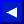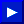the numbers are rounded to nine digits when results are printed. Some examples of simple floating-point numbers are:

1.23
-.998877
+3.1459
.7777777
-333.
.01

Numbers smaller than .01 or larger than 999999999. will be printed in scientific notation. In scientific notation a floating-point constant is made up of three parts:

1. THE MANTISSA
2. THE LETTER E
3. THE EXPONENT

The mantissa is a simple floating-point number. The letter E is used to tell you that you're seeing the number in exponential form. In other words E represents * 10 (eg., 3E3 = 3*10^3 = 3000). And the exponent is what multiplication power of 10 the number is raised to.

Both the mantissa and the exponent are signed (+ or -) numbers. The exponent's range is from -39 to +38 and it indicates the number of places that the actual decimal point in the mantissa would be moved to the left (-) or right (+) if the value of the constant were represented as a simple number.

There is a limit to the size of floating-point numbers that BASIC can handle, even in scientific notation: the largest number is +1.70141183E+38 and calculations which would result in a larger number will display the BASIC error message ?OVERFLOW ERROR. The smallest floating-point number is +2.93873588E-39 and calculations which result in a smaller value give you zero as an answer and NO error message. Some examples of floating-point numbers in scientific notation (and their decimal values) are:

 0.235988 (.235988) 2.359e+09 (2359000000.) -7.09e-12 (-.00000000000709) -314159 (-314159.)

String constants are groups of alphanumeric information like letters, numbers and symbols. When you enter a string from the keyboard, it can have any length up to the space available in an 80-character lineThis page has been created by Sami Rautiainen. Read the small print. Last updated November 13, 1998.